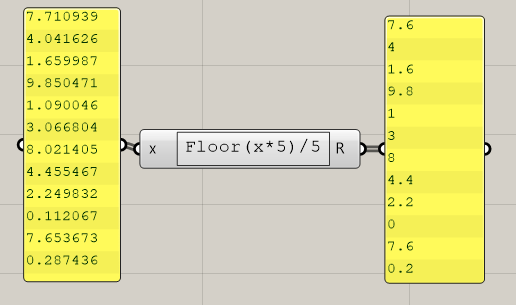# Round to nearest but always to smaller value

Hello,

I need to figure out how i can round numbers to the closest value in the set but so that it is always rounded to the smaller value.

so if i have 9,55 and possible values are 9,4 and 9,6 so resulting rounded number will be 9,4 and not 9,6

Here’s a way.

inset_below.gh (11.6 KB)

It’s a bit overcomplicated due to the fact that pufferfish is taking the value above in case one of your value is exactly between two values from your set.
In the inner group we check if by taking one index lower, we also get a difference of 0 (matching value). If it’s true, we offset the index by -1 (to get the lower of the two values in set that sit at the same distance from the tested value). sorry if badly explained, I could have another go if neededCheers !

Note: I see that I forgot to edit the description of the first group. It should now be correct.

1 Like

Here’s another easier approach using number domains:

round to lower.gh (12.8 KB)

Edit: One thing I forgot: The list of target values must be sorted ascending.

4 Likes

If you want to round down to the closest multiple of 0.2 below:6 Likes

You don’t even need the domains…

round to lower.gh (12.7 KB)

I think the floor trick by Daniel is the shortest and best answer here.

2 Likes

thank you guysdifferent approaches, the simplest one is the winner

What would need to be added to the expression so 4.041 is output as 4.0 instead of just 4?

You can use `Format`

Thanks Daniel. That’s what I did until @kev.r pointed out it can slow down a definition…